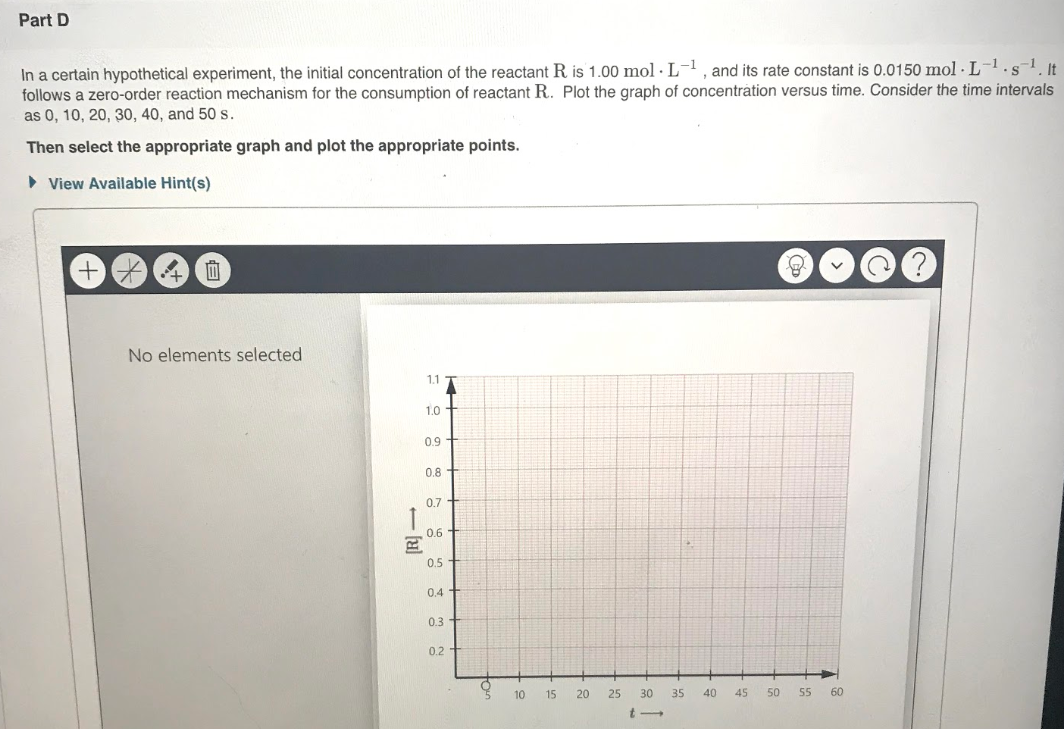# In a certain hypothetical experiment, the initial concentration of the reactant R is 1.00 mol • L ^ -1, and its rate constant is 0.0150 mol • L^-1 • s. It follows a zero-order reaction mechanism for the consumption of reactant R. Plot the graph of concentration versus time. Consider the time intervals as 0, 10, 20, 30, 40, and 50 s. Then select the appropriate graph and plot the appropriate points.